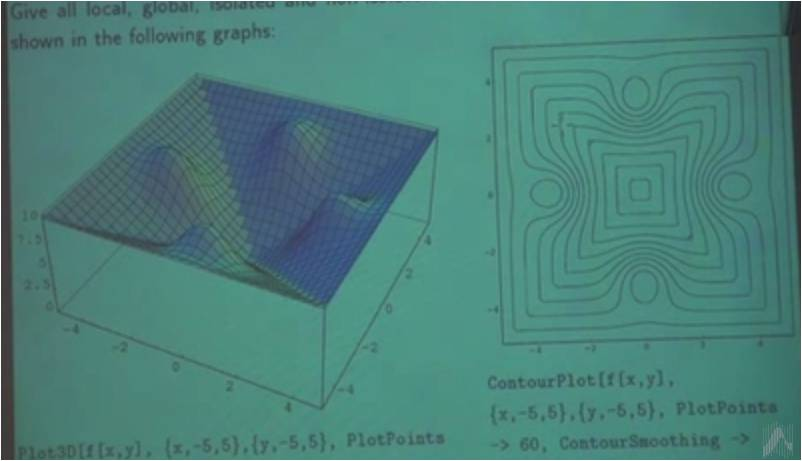# InfoCoBuild

## Advanced Mathematics for Engineers 1

Advanced Mathematics for Engineers 1 (Hochschule Ravensburg-Weingarten Univ.). Instructor: Professor Wolfgang Ertel. After a repetition of basic linear algebra, computer algebra and calculus, this course will deal with numerical calculus, statistics and function approximation, which are the most important basic mathematics topics for engineers in the fields of computer science, mechatronics and electrical engineering.

Topics covered in this course, Advanced Mathematics for Engineers 1, include: computer algebra; calculus including sequences, power series, continuity, Taylor series, and differential calculus in many variables; discrete distributions; roots of nonlinear equations, and method of least squares and pseudoinverse.Lecture 01 - Computer Algebra Lecture 02 - Sequences, Introduction to Mathematica Lecture 03 - Introduction to Octave, Series Lecture 04 - Power series, Continuity, Discontinuity Lecture 05 - Continuity, Discontinuity, Taylor Series Lecture 06 - Differential Calculus in Many Variables Lecture 07 - The Total Differential, Extrema without/with Constraints Lecture 08 - Extrema, Statistics and Probability Lecture 09 - Discrete Distributions: Binomial Distribution, Hypergeometric Distribution Lecture 10 Lecture 11 Lecture 12 - Roots of Nonlinear Equations: Fixed Point Iteration, Banach Fixed Point Theorem Lecture 13 - Banach Fixed Point Theorem, Convergence Speed and Convergence Rate, Newton's Method Lecture 14 - Polynomial Interpolation, Spline Interpolation Lecture 15 - Spline Interpolation: Correctness and Complexity, Interpolation of Arbitrary curves Lecture 16 - Method of Least Squares and Pseudoinverse Lecture 17 - Method of Least Squares and Pseudoinverse: Multidimensional Least Squares Lecture 18 - Application of the Pseudoinverse for Function Approximation

 References Advanced Mathematics for Engineers | Hochschule Ravensburg-Weingarten Instructor: Professor Wolfgang Ertel. Demos and Course Material. Octave Exam Programs. Previous Examinations. Video Lectures from Previous Semesters.